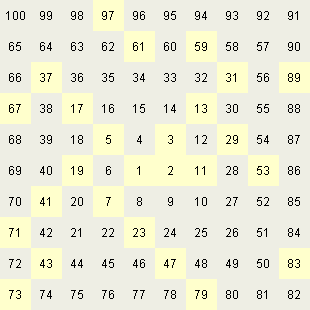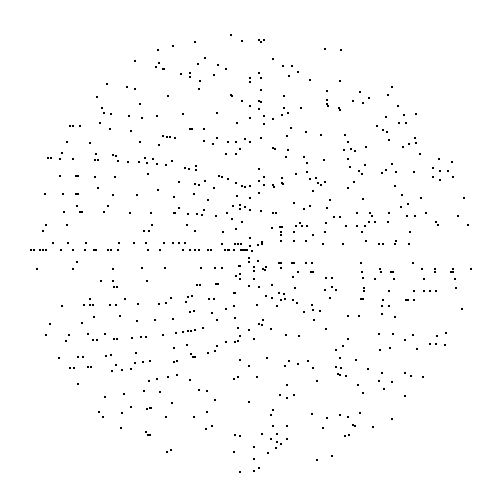NumberSpiral.com

CONTENTS7.  Primes

 Now that we've adopted a convention for naming the curves on the number spiral, let's look again at how primes are distributed. As shown in Figure 1, the densest concentrations of primes seem to occur mainly in curves whose offsets are prime.Figure 1

 When we look at a graph that shows only primes, like Figure 1, the left-facing curves are much more pronounced than the right-facing curves. However, when we look at a graph that shows all the integers, like Figure 3, the left-facing and right-facing curves are equally salient. The main reason for this seems to be that on the left side, primes can occur only in curves with odd offsets. On the right side, primes can occur in curves with both odd and even offsets. It would be interesting to investigate empirically whether equal quantities of primes occur on both sides. The number spiral is closely related to the Ulam prime spiral, shown in Figure 2:Figure 2

 Each diagonal of the Ulam spiral corresponds to a particular curve on the number spiral. However, the Ulam spiral makes two diagonals out of each curve by allowing both ends of a diagonal to grow in opposite directions and placing alternate members of the sequence at either end. Moreover, the two halves of each diagonal do not usually line up with each other. This sounds terribly confusing in words, but as shown in Figure 3, it's really pretty simple. I've labeled only a few diagonals in Figure 3 to illustrate the pattern, the correspondence extends infinitely:Figure 3Many pairs of prime numbers are separated by a single non-prime; for example, 29 and 31, or 41 and 43. Figure 4 shows the distribution of these twins (to be precise, it shows the intermediary non-primes that separate the twins) up to 49,284:Figure 4Back  1  2  3  4  5  6  7  8  9  10  Next